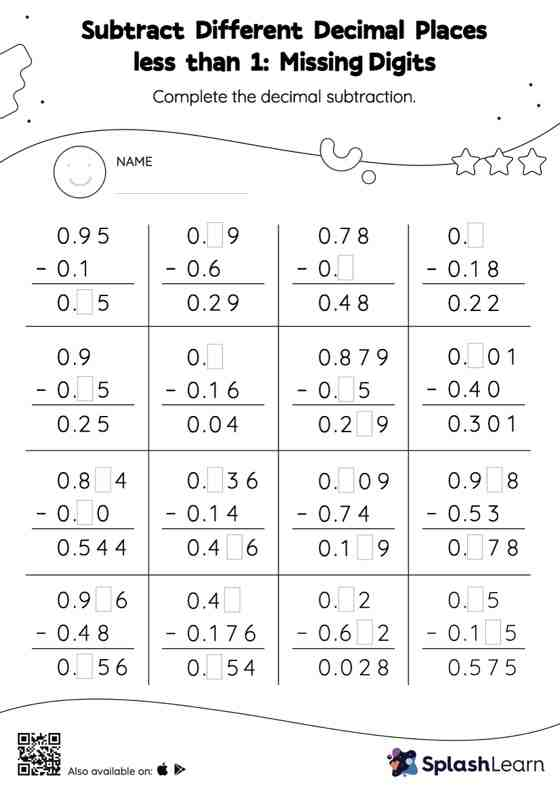# Subtract Different Decimal Places less than 1: Missing Digits Worksheet

Home > Subtract Different Decimal Places less than 1: Missing DigitsIn this worksheet, students subtract decimals by aligning the decimal points and using zero as a placeholder. They then use the relationship between addition and subtraction to find the missing number in the subtract different decimal places less than 1 worksheet. In each problem, the numbers are laid out in the vertical format. In this format, pair of digits in each successive place are tackled one by one. This helps students follow a structured approach.